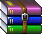• python好玩的代码-好玩的游戏
千次阅读
2020-10-30 23:04:23

[python]代码库import pygame

from random import randint

import math

print("按下空格建发射子弹")

pygame.init()

screen = pygame.display.set_mode((800, 600))

pygame.display.set_caption("飞机大战")

pygame.display.set_icon(icon)

pygame.mixer.music.play(-1)

bomb_sound = pygame.mixer.Sound('exp.wav')

playerX = 400

playerY = 500

playStep = 0

score = 0

font = pygame.font.Font('freesansbold.ttf', 35)

def show_score():

text = f"score:{score}"

score_render = font.render(text, True, (0, 200, 255))

screen.blit(score_render, (10, 10))

is_over = False

over_font = pygame.font.Font('freesansbold.ttf', 64)

def check_is_over():

if is_over:

text = "Game Over"

render = over_font.render(text, True, (255, 50, 10))

screen.blit(render, (230, 250))

number_of_enemies = 10

class Enemy():

def __init__(self):

self.x = randint(100, 600)

self.y = randint(50, 250)

self.step = randint(2, 5)

def reset(self):

self.x = randint(100, 600)

self.y = randint(50, 250)

Enemies = []

for i in range(number_of_enemies):

Enemies.append(Enemy())

def show_enemy():

global is_over

for e in Enemies:

screen.blit(e.img, (e.x, e.y))

e.x += e.step

if (e.x > 736 or e.x < 0):

e.step *= -1

e.y += 40

if e.y > 450:

is_over = True

print("游戏结束啦！")

Enemies.clear()

def distance(bx, by, ex, ey):

a = bx - ex

b = by - ey

return math.sqrt(a*a + b*b)

bullets = []

class Bullet():

def __init__(self):

self.x = playerX

self.y = playerY + 10

self.step = 10

def hit(self):

global score

for e in Enemies:

if (distance(self.x, self.y, e.x, e.y) < 40):

bomb_sound.play()

bullets.remove(self)

e.reset()

score += 1

def show_bullet():

for bull in bullets:

screen.blit(bull.img, (bull.x, bull.y))

bull.hit()

bull.y -= bull.step

if bull.y < 0:

bullets.remove(bull)

def distance_two(bx, by, ex, ey):

a = bx - ex

b = by - ey

return math.sqrt(a*a + b*b)

bullets_two = []

class Bullet_two():

def __init__(self):

self.x = playerX + 30

self.y = playerY + 10

self.step = 10

def hit_two(self):

for d in Enemies:

if (distance_two(self.x, self.y, d.x, d.y) < 40):

bomb_sound.play()

bullets_two.remove(self)

d.reset()

def show_bullet_two():

for b in bullets_two:

screen.blit(b.img, (b.x, b.y))

b.hit_two()

b.y -= b.step

if b.y < 0:

bullets_two.remove(b)

def move_player():

global playerX

playerX += playStep

while playerX > 736:

playerX -= 6

while playerX < 0:

playerX +=6

running = True

while running:

screen.blit(bgimg, (0, 0))

show_score()

for event in pygame.event.get():

if event.type == pygame.QUIT:

running = False

if event.type == pygame.KEYDOWN:

if event.key == pygame.K_RIGHT:

playStep = 6

elif event.key == pygame.K_LEFT:

playStep = -6

elif event.key == pygame.K_SPACE:

print("发射子弹....")

bullets.append(Bullet())

bullets_two.append(Bullet_two())

if event.type == pygame.KEYUP:

playStep = 0

screen.blit(playerimg, (playerX, playerY))

move_player()

show_bullet()

show_bullet_two()

show_enemy()

check_is_over()

pygame.display.update()

[源代码打包下载]faijidazan.zip(50积分)[26 次下载]更多相关内容
• python有趣的一行代码程序员式表白print(' '.join([''.join([('AndyLove'[(x-y)%8]if((x*0.05)**2+(y*0.1)**2-1)**3-(x*0.05)**2*(y*0.1)**3

python有趣的一行代码

程序员式表白

print(' '.join([''.join([('AndyLove'[(x-y)%8]if((x*0.05)**2+(y*0.1)**2-1)**3-(x*0.05)**2*(y*0.1)**3<=0 else' ')for x in range(-30,30)])for y in range(15,-15,-1)]))一行显示乘法表

print(' '.join([' '.join(['%s*%s=%-2s' % (y,x,x*y) for y in range(1,x+1)]) for x in range(1,10)]))一行显示迷宫

print(''.join(__import__('random').choice('╱╲') for i in range(50*24)))一行代码输出Mandelbrot图像

print(' '.join([''.join(['*'if abs((lambda a:lambda z,c,n:a(a,z,c,n))(lambda s,z,c,n:z if n==0else s(s,z*z+c,c,n-1))(0,0.02*x+0.05j*y,40))<2else' 'for x in range(-80,20)]) for y in range(-20,20)]))一行代码计算出1-100之间的素数

print(' '.join([str(item) for item in filter(lambda x: all(map(lambda p: x % p!= 0, range(2, x))), range(2, 101))]))一行代码输出前100项斐波那契数列的值

print([x for x in [ (a[i], a.append((a[i], a[i]+a[i]))) for a in ([[1,1]], ) for i in range(100) ]])展开全文• 前言：Python可谓是现在很多...用 50 行 Python 代码完成图片转字符画小工具。通过实验将学习到 Linux 命令行操作，Python 基础，pillow 库的使用，argparse 库的使用。用Python Turtle 模块，通过画出分形树（Frac...

前言：

Python可谓是现在很多人正在学或者想学的一个脚本语言了，提到学习自然就少不了拿项目练手，可是一般的项目根本提不起兴趣嘛，这10个项目可是非常有趣的，不信你看看。

用 50 行 Python 代码完成图片转字符画小工具。通过实验将学习到 Linux 命令行操作，Python 基础，pillow 库的使用，argparse 库的使用。用Python Turtle 模块，通过画出分形树（Fractal tree），科赫雪花曲线（Koch snowflake）和龙形曲线（Dragon curve）这三种曲线来加强对递归的认识。使用 Python3 去识别图片是否为色情图片，我们会使用到 PIL 这个图像处理库，会编写算法来划分图像的皮肤区域。其中涉及到Python 3 基础知识，肤色像素检测与皮肤区域划分算法，Pillow及argparse的使用。使用Python脚本爬取某租房网站的房源信息，利用高德的 js API 在地图上标出房源地点，划出距离工作地点1小时内可到达的范围。在项目实现的过程中熟悉了requests、BeautifulSoup、csv等库的简单使用。使用 Python3 抓取 12306 网站信息提供一个命令行的火车票查询工具。通过该项目的实现，可以熟悉 Python3 基础及网络编程，以及 docopt，requests，prettytable 等库的使用。使用 OpenCV 处理图片视频，将视频转为字符画序列，再在终端中播放字符动画。除了 OpenCV 的操作，本课程还会了解光标定位转义编码的使用。’哲学家就餐"’问题是计算机中的一个经典问题，用来演示在并发计算中多线程同步时产生的问题。该课程通过三种不同的方式解决"’哲学家就餐"’问题。加深对线程、死锁、活锁的认识。带领大家一步一步攻克Python Challenge中的难题，在一个又一个脑洞大开的 Python 问题中提升自己的 Python 水平。项目通过 OpenCV 库来实现人脸面部特征交换，其实就是将第二张人脸的眼睛、鼻子和嘴巴通过程序自动裁剪适配并覆盖到第一张人脸上，并且为了使得修改后的照片看着更加自然，我们还需要调整皮肤颜色。通过借助 docopt 库与 Pillow 库来构建命令行解析器获取指定图像并将其转换成相应样式的彩色字符图像，并在终端上将转化后的图像打印出来。大家可尝试选择自己喜欢的头像图片制作自己的彩色字符头像哦！后记：

上面介绍的10个Python项目是不是非常有趣！！开发着有趣的项目学习起来也觉得更加的容易。

如果你还想查看更多的Python项目，点击：

展开全文• 可能大家在网上已经看过类似的python单行代码可以实现的功能,比如：九九乘法表：print(' '.join([' '.join(['%s*%s=%-2s'%(y,x,x*y)for y in range(1,x+1)]) for x in range(1,10)]))或者输出前100项斐波那契数列的...可能大家在网上已经看过类似的python单行代码可以实现的功能,比如：

九九乘法表：

print(' '.join([' '.join(['%s*%s=%-2s'%(y,x,x*y)for y in range(1,x+1)]) for x in range(1,10)]))或者输出前100项斐波那契数列的值：

print([x for x in [ (a[i], a.append((a[i], a[i]+a[i]))) for a in ([[1,1]], ) for i in range(100) ]])嗯，这些都可以做到，那么下面的这些炫酷的代码，更值得一看！

单线迷宫（cmd下输入）：

python -c "while 1:import random;print(random.choice('|| __'), end='')"emmmm，这个可能比较抽象，那么试试将里面的字符换一下，比如下面这个代码python -c "while 1:import random;print(random.choice('╱╲'), end='')"这个迷宫效果还可以吧！

猜数字

好多的教程中都有这个小案例，那么你用了几行呢？试试下面这个

python -c "import random;n=random.randint(1,99);[(lambda a:print('Y' if a==n else 'H' if a>n else 'L'))(int(input())) for i in range(6)]"是的，你没有看错，一行代码可以实现这个功能！

100英尺高尔夫

参照了"愤怒的小鸟”，目标是让高尔夫刚好落到100英尺处，不多不少！

import math as m;a,v=eval(input());[print('%03d'%x+' '*m.floor(0.5+x*m.tan(a)-x*x/(v*m.cos(a)))+'o') for x in range(102)]需要输入2个参数，也就是高尔夫的角度和速度！

最后

这些代码使用的大多数技术应该看起来很熟悉:

列表解析，lambda表达式和随机整数，通过几个函数的组合，可以写出很酷的代码！

展开全文• # coding=utf-8Version: Python3.7.4Author: SimonSite: https://gitee.com/simon4055Time: 2019/08/07 18:00user_agent_list = ["Mozilla/5.0 (Windows NT 6.1; WOW64) AppleWebKit/537.1 (KHTML, like Gecko)...
• 这是本人在学习Python时候自己写的好玩的Python程序，里面包含了可以说是python基础的全部吧，非常的适合小白学习Python，谢谢大佬了~~~
• 其实Python是入门容易精通难，大家平时要养成记录的习惯，好的代码要记录下来，学会总结，经常翻一翻会有收获！今天我来分享一些我自己记录的代码结构，欢迎拍砖！01.简洁的表达式点评：Python因为简洁高效而出名，...
• Python是一门清晰易学的语言，可是这不代表我们Python没有...以下代码如果不信请手动尝试，测试环境：Python 3.620跟21有什么区别第一次看到这个例子的时候我的心里面充满了wtf，20跟21有什么区别为什么结果不一样？...
• 先选取了 6 个自己认为值得玩味的 python 代码，希望对正在学习 python 的你有所帮助。1、类有两个方法，一个是 __new__,一个是 __init__,有什么区别，哪个会先...列表 java 大数据 编程语言
• 逛 GitHub 的时候，发现了一个很酷的 Python 训练项目集。一共有 25 个题目，基本涵盖了用 Python 实现的各种功能。上一周没有复习 C++ 和网络通信全部用来玩这几个题了。题目地址在 –> GitHub | Dumb Ways To Code...
• image.png简单的说,GUI编程就是给程序加上图形化界面.python的脚本开发简单,有时候只需几行代码就能实现丰富的功能,而且python本身是跨平台的,所以深受程序员的喜爱.如果给程序加一个图形化界面,那么普通的用户也就...
• 然后马上上电脑打上3组趣味代码 import time words = input('Please input the words you want to say!:') for item in words.split(): print('\n'.join([''.join([(item[(x-y) % len(item)] if ((x*0.05...开发语言 后端
• 本实验将使用 Python3 去识别图片是否为色情图片，我们会使用到 PIL 这个图像处理库，会编写算法来划分图像的皮肤区域 1.1. 知识点 Python 3 的模块的安装 Python 3 基础知识 肤色像素检测与皮肤区域划分算法 Pillow...
• 前言 作者 ：“程序员梨子” **文章简介 **：本篇文章主要是写了利用Turtle库绘制四种不一样的图案的小程序！...Turtle库是Python语言中一个很流行的绘制图像的函数库，想象一个小乌龟，在一个横轴为x、纵程序员 Turtle
• 最近在做Python项目开发的时候愣是发现了好多好玩的小项目，并且都是代码量较少容易上手的，所以今天就来和小伙伴分享一些Python从一行代码到三十行代码都有哪些好玩的项目。之后大灰狼也会在这里持续更新更多好玩的...人工智能 深度学习 程序人生
• Python实现在微信头像右上角加上红底白字的数字，类似于微信未读信息数量那种提示效果
• Python 能做很多无聊，但有意思的事情，例如接下来的一些案例。 Python 整蛊程序 以下程序，不要发代码，要不实现不了你整蛊的目的。 要打包成一个 exe 程序，发给朋友才有意思。 使用 pip install pyinstaller。 ...开发语言
• 一些复杂的任务，甚至可以使用一行Python代码就能搞定。 下面，我给大家介绍50个有趣的Python一行代码，都很实用。 希望大家能从中找到对自己有帮助的技巧。 ▍1、字母异位词 两个单词如果包含相同的...算法
• 注意：我用的python2.7，大家如果用Python3.0以上的版本，请记得在print()函数哦！如果因为版本问题评论的，不做回复哦！！！ 1.题目：有1、2、3、4个数字，能组成多少个互不相同且无重复数字的三位数？都是多少？ ...
• Python是一门非常简洁而优美的编程语言，在其他编程语言中需要繁琐的代码逻辑才能完成的事情，往往在Python中一行就可以解决。这样，它不仅可以减少开发工作量，还能够大大提升可读性，避免在开发复杂逻辑过程中造成...
• # coding=utf-8Version: Python3.7.4Author: SimonSite: https://gitee.com/simon4055Time: 2019/08/07 18:00user_agent_list = ["Mozilla/5.0 (Windows NT 6.1; WOW64) AppleWebKit/537.1 (KHTML, like Gecko)...
• 前言本月将更新八篇python有趣系列文章。 本系列通过多个有趣案例，讲解python的玩法，其中包含如下内容，一一推进讲解。 爬虫数据分析机器学习项目背景最近知乎老是给我推送两个问答，一个是长得好看是种什么体验？...
• Python”编程语言的学习兴趣，今天给大家分享几款有趣的Python程序代码，感兴趣的小伙伴可以跟着学习借鉴哦!qq进群：156846986可以获取Python学习资料哦！https://www.wepeng.net/course/detail/3/用Python代码创作...
• 立即注册 x为提高大家对“Python”编程语言的学习兴趣，今天给大家分享几款有趣的Python程序代码，感兴趣的小伙伴可以跟着学习借鉴哦!进群：839383 765可以获取Python学习资料哦！分享一："啥是佩奇?"让Python语言...
• 不要害怕学习的过程枯燥无味，这里有程序员 jackzhenguo 打造的一份中文 Python “糖果包”：147 个代码小样，60 秒一口，营养又好玩，从 Python 基础到机器学习尽皆囊括。入门简单如十进制转二进制，尽显 Python ......